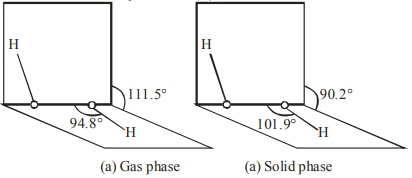# Given below are two statements: One is labelled as AssertionQuestion:

Given below are two statements: One is labelled as Assertion $\mathbf{A}$ and other is labelled as Reason $\mathbf{R}$. Assertion A : The dihedral angles in $\mathrm{H}_{2} \mathrm{O}_{2}$ in gaseous phase is $90.2^{\circ}$ and in solid phase is $111.5^{\circ}$. Reason $\mathbf{R}$ : The change in dihedral angle in solid and gaseous phase is due to the difference in the intermolecular forces.

Choose the most appropriate answer from the options given below for $\mathbf{A}$ and $\mathbf{R}$.

1. $\mathbf{A}$ is correct but $\mathbf{R}$ is not correct.

2. Both $\mathbf{A}$ and $\mathbf{R}$ are correct but $\mathrm{R}$ is not the correct explanation of $\mathrm{A}$.

3. Both $\mathbf{A}$ and $\mathbf{R}$ are correct and $\mathbf{R}$ is the correct explanation of $\mathbf{A}$.

4. $\mathbf{A}$ is not correct but $\mathbf{R}$ is correct.

Correct Option: , 4

Solution:(a) $\mathrm{H}_{2} \mathrm{O}_{2}$ structure in gas phase, dihedral angle is $111.5^{\circ}$. (b) $\mathrm{H}_{2} \mathrm{O}_{2}$ structure in solid phase at $110 \mathrm{~K}$, dihedral angle is $90.2^{\circ}$.

Hence given statement (A) is not correct But statement (B) is correct.